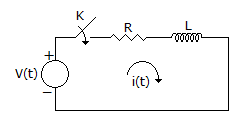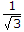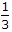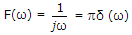# Electronics and Communication Engineering - Networks Analysis and Synthesis

1.

In the circuit shown in the given figure, switch k is closed at t = 0. The circuit was initially relaxed then which one of the following sources of v(t) will produce maximum current at t = 0+?A. Unit step B. Unit impulse C. Unit ramp D. Unit step plus unit ramp

Explanation:

No answer description available for this question. Let us discuss.

2.

Pick the correct statement

 A. Delta connection draws same current as star connection B. Delta connection draws 3 times as much current as star connection C. Delta connection drawsof the current of star connection D. Delta connection drawsof the current of delta connection

Explanation:

No answer description available for this question. Let us discuss.

3.

The inverse Fourier transform ofis

 A. sin ωt B. cos ωt C. sgn (t) D. u (t)

Explanation:

No answer description available for this question. Let us discuss.

4.

The colour on the extreme left in general purpose fixed resistors represents

 A. first significant digit B. tolerance C. wattage rating D. voltage rating

Explanation:

No answer description available for this question. Let us discuss.

5.

When a current flows through a conductor,

 A. an electric field exists at all points within the conductor B. electric field exists only at all points on the surface of the conductor C. electric field exists only at ends of the conductor D. any of the above depending on magnitude of current